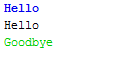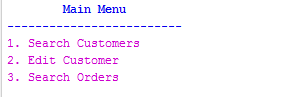# Python: Variables and Output

Creating basic Python programs begins with basic output and variables.

• Output is accomplished with the print command.
• Variables are used to store data and are created by giving them a name and assigning them a value.

#### Example: Python Output

Below is how easy it is to create a very simple Python program. This is the classic "Hello World" program that is used in many programming language as the first program one learns to write. The syntax of Python is very simple, which is a major reason for the language's popularity.

This program is outputting a string. This is evident because of the quotation marks.

``````
print("Hello world")
``````

Output:

Hello World!

#### Example: Python Output

This program is outputting a number. This is evident because there are no quotation marks. A number can be an integer (e.g. 1, 200, 303, etc.) or a float (e.g. 332.323, 2.3, -1.2, etc.)

``````
print(9)
``````

Output:

9

#### Example: Python Output

Below is how easy it is to create a very simple Python program. This is the classic "Hello World" program that is used in many programming language as the first program one learns to write. The syntax of Python is very simple, which is a major reason for the language's popularity.

This program is outputting a string. This is evident because of the quotation marks.

``````
print("Hello world")
``````

Output:

Hello World!

#### Example: Python Output - Multiple lines

It is equally easy to output more than one line as shown below.

``````
print("Two roads diverged in a yellow wood,")
print("And sorry I could not travel both")
print("And be one traveler, long I stood")
``````

Output:

Two roads diverged in a yellow wood,
And sorry I could not travel both
And be one traveler, long I stood

#### Example: Simple math in Python

It is very easy to do math in Python.

``````
print(2 * 10)
``````

Output:

20

#### Example: Python Output of Strings and Variables

If you want to store data in a program you use variables. Data in Python is most often one of three data types:

• Integer, e.g. 1, 20, 25, 100, etc.
• Float, e.g. 10.5, .99, 920.4343, etc.
• String, Hello, Today, etc.

In the example below, two variables are created: x and y. They are also assigned values: 45 and 19.5. Their data type is integer and string respectively. The third line prints a string Hello World!. The last line prints the x = and then value stored in x and then y = and the value stored in y.

The pound symbol (#) is the comment (remark) symbol in Python. The use of multiple #### symbols below was for visual purposes only.

``````
#############################################################
# Author:       John Smith
# Filename:     output1.py
# Description:  Program to demonstrate basic output in Python
#############################################################
x = 45
y = 19.5
print ("Hello, world!")
print ("x =", x, "and y =", y)
``````

Output:

Hello World!
x = 45 and y = 19.5

#### Example: More on Variables

You can overrite the value stored in a variable.

``````
age = 25
age = 35
print(age)
age = 45
print(age)
``````

Output:

35
45

#### Example: Python Output - comma vs. plus sign

You can seperate items to be printed by a comma or plus sign. The comma adds a space; the plus sign does not.

``````
message1 = "Hello"
message2 = "Goodbye"
print (message1,message2)
print (message1 + message2)
``````

Output:

Hello Goodbye
HelloGoodbye

#### Example: Formatting Numbers

The example below demonstrates several ways to format number output in Python. There are many additional ways to do so as well. The % symbold used this way is a modulo operator.

``````
salary = 35500
print("Salary: ", salary)
print("Salary: %.2f" % salary)
print("Salary: \$%.2f" % salary)
print("Salary: \${:,.2f}".format(salary))
``````

Output:

Salary: 35500
Salary: 35500.00
Salary: \$35500.00
Salary: \$35,500.00

#### Example: Formatting Numbers

``````
x = 10.532
y = 11

print(x,y)

print("x = ", x)
print("x = %d" % x)    # display as signed integer decimal
print("x = %5d" % x)   # allocated five spaces for number
print("x = %.1f" % x)  # display one digit to right of decimal

print("x = ", y)
print("x = %.2f" % y)
print("x = %8.2f" % y)  # allocate 8.2 spaces
``````

Output:

10.532 11
x =     10.532
x = 10
x =      10
x = 10.5
x =   11
x = 11.00
x =    11.00

#### Example: Escape Codes

Like many other programming languages, Python supports escape codes. The example below demonstrates several of these.

• \t is the tab code (causea a tab, i.e. about 5 spaces
• \n print a new (blank) line
• end="" prevents a new line break.
``````
print("Column 1\tColumn 2")
print("\n\n")
print("William ", end="")
print("Susan ", end="")
``````

Output:

Column 1        Column 2

William Susan

#### Example: Splitting Strings

The example below demonstrates how to split strings.

``````
# Python Splitting Strings Example Program
# February 20, 2020
# Author: Code ABC's
EmpName1 = "John Smith"
print("Employee 1 name: ", EmpName1)
print("Employee 1 name: ", EmpName1[0:4])
print("Employee 1 name: ", EmpName1[5:])
print("Employee 1 name: ", EmpName1[5:] + ", " + EmpName1[0:4])
``````

Output:

Employee 1 name: John Smith
Employee 1 name: John
Employee 1 name: Smith
Employee 1 name: Smith, John

#### Example: How to Format Numbers

Open in new window

#### Example: Example of Constants in Python

``````
# Example of a constant in Python
TAX_RATE = .25

gross_salary = 49000
after_tax_salary = gross_salary * (1 - TAX_RATE)

print("Your after tax salary is %.2f" % after_tax_salary)
``````

Output:

Your after tax salary is 36750.00

#### Example: Swapping numbers

``````
x = 100
y = 200
y,x = x,y

print(x)
print(y)
``````

Output:

200
100

#### Example: More Basic Math in Python

``````
# Basic temperature conversation
f = 70
c = (f - 32) * 5 / 9
print(f, "F equals", c, "C.")
print(f, "F equals %.0f " % c + "C.")
``````

Output:

70 F equals 21.11111111111111 C.
70 F equals 21 C.

#### Example: Multiplying characters

``````
print("----------")
print("-" * 10)
``````

Output:

----------
----------

#### Example: Outputting multiple arguments

``````
x, y, z = 15.5555, 20, 44.3
print("The variable values are %.2f %.2f %.2f" % (x, y, z) + ".")
``````

Output:

The variable values are 15.56 20.00 44.30.

#### Example: Math operation that divides and provides the integer/floor result

``````
x = 9
y = 9 // 4
print(y)
``````

Output:

2

#### Example: Using Colors and Other Effects in Output

``````
BLUE  			= "\033[1;34m"
LIGHTBLUE		='\033[94m'
CYAN  			= "\033[1;36m"
LIGHTCYAN		='\033[96m'
RED   			= "\033[1;31m"
GREEN 			= "\033[0;32m"
PURPLE			='\033[35m'
BOLD    		= "\033[;1m"
STRIKETHROUGH	='\033[09m'
RESET 			= "\033[0;0m"

print(BLUE + "Hello", RESET)
print("Hello")
print(GREEN + "Goodbye", RESET)
``````

Output:#### Example: Using Colors and Other Effects in Output

``````
BLUE    = "\033[1;34m"
PURPLE	='\033[35m'
RESET   = "\033[0;0m"

print(BLUE + "\t\tMain Menu")
print("-" * 25)
print(PURPLE + "1. Search Customers")
print("2. Edit Customer")
print("3. Search Orders", RESET)
``````

Output:Errors in programs and debugging

Programs may not work, or not work correctly, for a variety of reasons. There are two main types of errors in programs:

• Syntax error - an error in the syntax (or grammar) of the language/program. For example, mistyping or a keyword/command or not including a required comma or double quote. Syntax errors often cause the program not to run and an error message to display instead.
• Semantic - an error in the logic or algorithm of the program. Programs with a semantic may still run, but not run correctly or produce the desired output.

#### Example: Basic Input and Output

In a future section, we will explore how to allow the user to provide the program input. For a quick look at this, you can view this video with a simple example of it.

### Quiz

1. Which type of error is one that causes a program not to compile or run. For example, misspelling a command may cause this. (choose best answer)
Syntax
Minor
Trace
Semantic

2. What of the following Python commands is invalid?
print("hello world")
print(hello world)
print(9)
print(9-2)

3. What the output of this program

``````
print(4*4+2)
``````
4*4+2
24
10
18

4. What Python data type is this:    4.54    ?
Float
String
Integer
Semantic

5. What the output of this program

``````
x = 209.1234567
print("%.3f" % x)
``````
209.1234567
209.123
209.1234
3 209.1234567

6. Which of the following used in a print statement will output a tab (i.e. about 5 spaces)?
\tab
/tab
\t
\tab

7. What the output of this program

``````
print("^" * 5)
``````
^5^
15
^ 5
^^^^^

8. What the output of this program

``````
# Hello World
age = 15
``````
15
Hello World
# Hello World
* There will be no output to this program